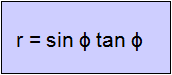# cissoid

## cubicThe cissoid 1) can be constructed as follows: Given a circle C with diameter OA and a tangent l through A. Now, draw lines m through O, cutting circle C in point Q, line l in point R. Then the cissoid is the set of points P for which OP = QR.This construction has been done by the Greek scholar Diocles (about 160 BC) 2). He used the cissoid to solve the Delian problem, dealing with the duplication of the cube. He did not use the name cissoid. The name of the curve, meaning 'ivy-shaped', is found for the first time in the writings of the Greek Geminus (about 50 BC). Because of Diocles' previous work on the curve his name has been added: cissoid of Diocles. Roberval and Fermat constructed the tangent of the cissoid (1634): from a given point there are either one ore three tangents. In 1658 Huygens and Wallis showed that the area between the curve and his asymptote is π/4. The cissoid of Diocles is a special case of the generalized cissoid, where line l and circle C have been substituted by arbitrary curves C1 and C2. The curve, having one cusp and one asymptote, has as Cartesian coordinates: x3 + xy2 - y2 = 0 or y2 = x3/(1-x) Another generalization of the curve is the ophiuride.Some relationships with other curves are: the curve is a pedal as well as the polar inverse (with the cusp as center of inversion) of the parabola its catacaustic (with the cusp as source) is the cardioid when a parabola is rolling over another (equal) parabola, then the path of the vertex is a cissoid its pedal (with the focus as pedal point) is the cardioid In the special case that construction line l passes through the center of the circle, the curve is a strophoid. And near the cusp the curve approaches the form of a semicubic parabola. notes 1) In Italian: cissoide di Diocle.2) Waerden 1950 p.297